## Overview

Option Kh (watt-hours per pulse or Kh Watthour Constant) specifies number of watt-hours that must accumulate for each pulse generated by the meter. Each pulse includes an ON (conducting) and OFF period. The number of watt-hours may be small, even less than one, or large; 1000 watt-hours (one kilowatt-hour) is a common choice.

## Specification

To order a WattNode® Pulse meter with Option Kh, you must also indicate the current rating of the current transformers (CTs) you are using with the meter. For example, the following sets the current transformer rated amps to 150 and the watt-hours per pulse to 100 Wh (0.1 kWh):

`Opt CT=150,Kh=100`

You may want to convert between Kh “watt-hours per pulse” and Pk “pulses per kilowatt-hour”. The following equations show the conversions.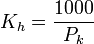$K_h = frac { 1000 } { P_k }$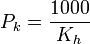$P_k = frac { 1000 } { K_h }$

For example, if you want 10 pulses per kilowatt-hour, you would solve for Kh as follows: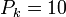$P_k = 10$ 10 pulses per kilowatt-hour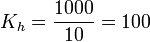$K_h = frac { 1000 } { 10 } = 100$ 100 watt-hours per pulse

## Option Hz Equivalence

Specifying Option CT and Option Kh is essentially equivalent to specifying a custom full-scale pulse frequency (Option Hz). It isn’t possible to specify both Option Hz and Option Kh at the same time. The following table contains equations to compute the full-scale frequency (Hz) values as a function of the specified CT amps and Kh (watt-hours per pulse) values.

Model Full-Scale Hz Equations
Standard Bidirectional Pulse Outputs
Option DPO (Dual Positive Outputs)
Option P3 (Per-Phase Outputs)
WNB-3Y-208-P
WNB-3D-240-P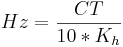$Hz=frac{CT}{10*K_h}$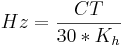$Hz=frac{CT}{30*K_h}$
WNB-3Y-400-P
WNB-3D-400-P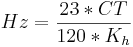$Hz=frac{23*CT}{120*K_h}$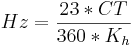$Hz=frac{23*CT}{360*K_h}$
WNB-3Y-480-P
WNB-3D-480-P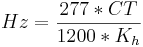$Hz=frac{277*CT}{1200*K_h}$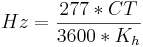$Hz=frac{277*CT}{3600*K_h}$
WNB-3Y-600-P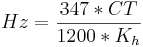$Hz=frac{347*CT}{1200*K_h}$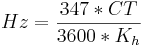$Hz=frac{347*CT}{3600*K_h}$

The general equation follows.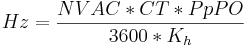$Hz=frac{NVAC*CT*PpPO}{3600*K_h}$

where,

• NVAC is the nominal line-to-neutral voltage
• CT is the current transformer rating in amps
• PpPO is phases per pulse output (as explained in the Power and Energy Equations section of the user manual)
• Kh is the watt-hours per pulse